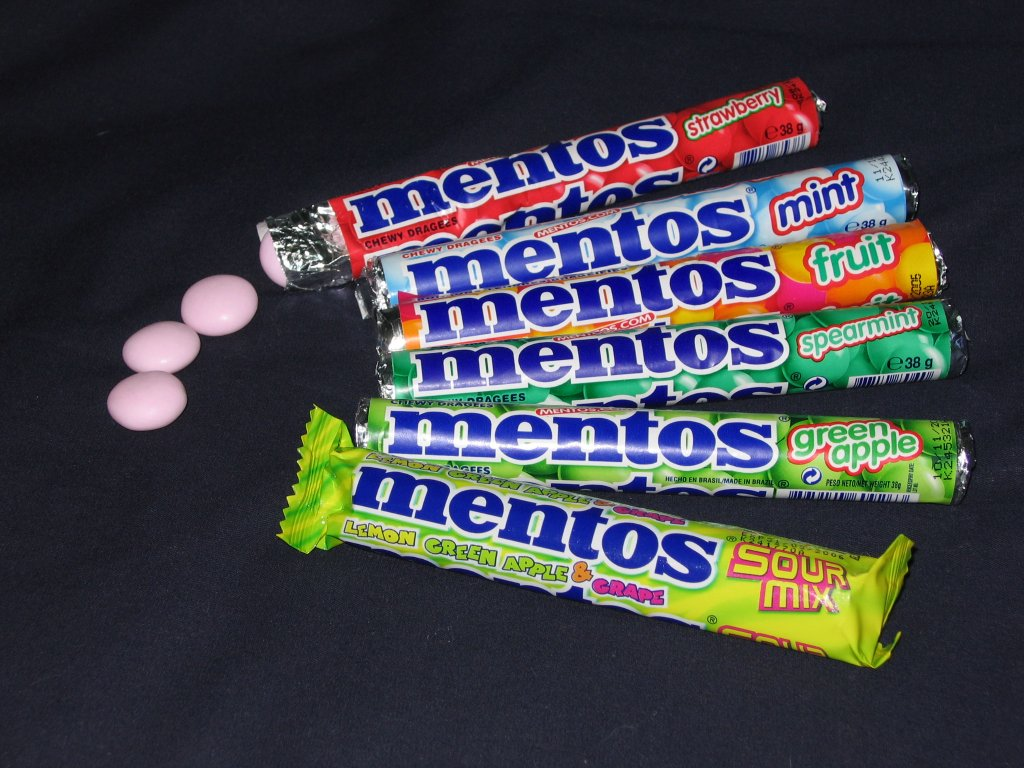## Notes on Representation – Multiplication

People who went through school in prior generations typically were sure that multiplying two quantities is harder to do than adding those same quantities.  My impression is that this perception hasn’t really changed with the wide-spread use of four-function calculators.  Even though on the four-function calculator$+$ and$\times$ are both single keystrokes that show instantaneous (or near-instantaneous) results, the memory of doing multi-digit multiplication in longhand in elementary school still lingers.

In previous posts I’ve played with the notion that these perceptions of “addition easy; multiplication hard” might have less to do with the nature of multiplication and more to do with the nature of our common system of representation of quantity, the (justly celebrated) decimal number system.  In particular, I’ve showed one way to represent quantity that is designed to make the act of multiplication really simple to do.  There are other representations, in wide practical use, that achieve the same thing.  For example, logarithmic charts for stock prices (e.g. here) allow you to see at a glance when prices are doubled, or cut in half, regardless of the starting price level.  In such a chart, the quantity (the price) is represented by a vertical line with a length proportional to the logarithm of the price.

Once we drop the notion that multiplication is inherently harder to do than addition, we have an opportunity to look freshly at the idea of multiplication to see if the idea is easy or hard.

A model that is often used to represent multiplication in the early grades – often the model through which multiplication is introduced – is that of certain numbers of items in a group and then having several groups.  If each roll of Mentos contains 14 candies, how many candies are there in 4 rolls?rolls of Mentos

The Mentos model can be usefully extended to a measurement model.  If one tassel is made of 7 inches of rope, how much rope would you need for 6 tassels?  This representation of multiplication allows for straight-forward extension to non-whole numbers: If one tassel is made of$7 \frac{3}{8}$ inches, how much rope would you need for 6 tassels?  Similarly, measurements in different units lead to multiplication:  If a stick is six feet long, and each foot is 12 inches, how long is the stick in inches?  Or: If a stick is six feet long, and each foot is 30.48 centimeters, how long is the stick in centimeters?

And then there is the area model.  If the bathroom is 21 tiles wide and 17 tiles long, how many tiles does it take to cover the bathroom floor?  this one leads itself well to non-whole numbers in both directions: If the bathroom is 20.5 tiles wide and 17.5 tiles long, how many tiles does it take to cover the bathroom floor?

In each of these models, the meaning of what is being asked is reasonably clear, even if a numerical answer to the question might not be on the tip of your tongue.  All of these models, in some ways, are models for multiplication.  Of course, there are many more.  An important group of models is based on stretching and scaling:I believe it is a mistake to argue about which of these models and representations (if any) is the real multiplication.  Each of them is – but each highlights some aspects and suppresses some others.

This entry was posted in Uncategorized and tagged , , , , . Bookmark the permalink.